﻿ 室外热环境指标的简化计算和应用研究
 快速检索 中文标题 英文标题 作者中文名 作者英文名 单位中文名 单位英文名 中文关键词 英文关键词 中文摘要 英文摘要 基金项目 全文
 气象2011, Vol. 37Issue (6): 701-706.### 引用本文 [复制中英文]

[复制中文]
CAI Ziying, HAN Suqin, ZHANG Changchun, et al, 2011. Simplified Method to Calculate Outer Thermal Environment Index and Its Application[J]. Meteorological Monthly, 37(6): 701-706. DOI: .
[复制英文]

### 文章历史

2010年5月12日收稿
2010年12月20日收修定稿

Simplified Method to Calculate Outer Thermal Environment Index and Its Application
, HAN Suqin, ZHANG Changchun, YAO Qing, HUANG He
Tianjin Institute of Meteorology, Tianjin 300074
Abstract: By using temperature, humidity, wind speed, wet bulb globe temperature (WBGT), and solar radiation data during May to September 2008 and May to July 2009 obtained from Tianjin boundary layer observation stations, through correlation analysis and multiple regression, a WBGT simplified calculation method is established. At the same time, by using Rayman model, based on the cloud cover the average radiation temperature is calculated, achieving the weather forecasting factors, combining with GB/T 17244-1998 and ISO 7243 standard systems, further using WBGT forecast products and giving a proposal about labor time arrangements in the next 6 hours, so as to reduce the injury from high temperature outdoor working. The results show that, the WBGT is highly related with dry-bulb temperature and solar radiation. Based on conventional meteorological data and average radiation temperature, the WBGT simplified calculation methods are practical and feasible. If the WBGT prediction products are applied to the future work schedule arrangements, there will be a better guide effect.
Key words: wet bulb globe temperature(WBGT)    average radiation temperature    labor time    Rayman model    forecasting factor

1 资料和方法 1.1 资料说明

 ${T_{{\rm{WBG}}}} = 0.7{T_s} + 0.2{T_g} + 0.1{T_a}$ (1)

1.2 模式和方法介绍 1.2.1 Rayman模型

Rayman模型是Andreas Matzarakiskai开发的热环境评估模型，模型以云、风、大气温度、相对湿度、水汽压等为输入因子，通过求解人体热量平衡方程，获取TPE(人体生理等效温度)、PMV(预计冷热感指标)、TSE(标准有效温度)等热环境评价因子。模型中平均辐射温度可以基于云量或太阳辐射观测资料进行计算

1.2.2 基于太阳辐射计算平均辐射温度

 ${T_{{\rm{mrt}}}} = {\left[{\frac{1}{\sigma }\sum\limits_{i = 1}^n {({E_i} + {a_k}\frac{{{D_i}}}{{{\varepsilon _p}}}){F_i}} } \right]^{0.25}}$ (2)

 ${T_{{\rm{mrt}}}}^{'} = {\left[{T_{_{{\rm{mrt}}}}^{^4} + {f_p}{a_k}\frac{{{I^*}}}{{{\varepsilon _p}\sigma }}} \right]^{0.25}}$ (3)

1.2.3 基于云量计算平均辐射温度

hsl≤4°时，

 ${R_{{\rm{prim}}}} = 1.4{(1.388-0.215{h_{{\rm{sl}}}})^2}(1-0.01{a_c})$ (4)

hsl＞4°, N≤4%时，

 ${R_{{\rm{prim}}}} = 1.4(-100.428 + 73.981{\rm{ln}}{h_{{\rm{sl}}}})(1-0.01{a_c})$ (5)

hsl＞4°, 20%＜N≤50%时，

 ${R_{{\rm{prim}}}} = 1.4{{\rm{e}}^{(5.383-16.072/{h_{{\rm{sl}}}}}})(1-0.01{a_c})$ (6)

hsl＞4°, 51%＜N≤80%时，

 ${R_{{\rm{prim}}}} = 1.4{{\rm{e}}^{(5.012-11.805/{h_{{\rm{sl}}}}}})(1-0.01{a_c})$ (7)

hsl＞4°, N＞80%时，

 ${R_{{\rm{prim}}}} = 0.9506h_{_{{\rm{sl}}}}^{^{1.039}}(1-0.01{a_c})$ (8)

 ${T_{{\rm{mrt}}}}^*= {\left[{\frac{{{R_{{\rm{prim}}}}}}{{5.39 \times {{10}^{-8}}}} + \left( {273 + t} \right)^4} \right]^{0.25}} -273.15$ (9)
2 结果分析 2.1 基于常规气象要素TWBG的简化算法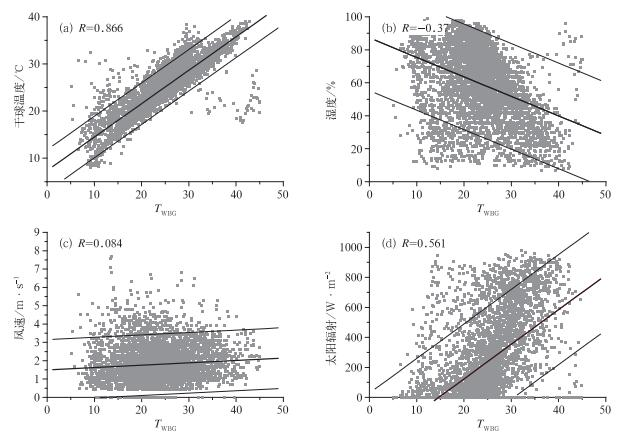图 1 干球温度(a)、湿球(b)、风速(c)、太阳辐射(d)与TWBG的关系 Fig. 1 The relation of (a) dry bulb temperature, (b) wet bulb temperature, (c) wind speed, and (d) solar radiation with TWBG
 ${T_{{\rm{WBG}}}} = 1.0031{T_a} + 0.021RH-0.083V + 0.002SR-2.822$ (10)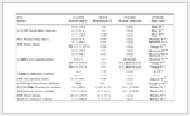表 1 基于常规气象要素回归模型的统计信息 Table 1 Regression model of statistical information based on the conventional meteorological elements表 2 基于常规气象要素回归系数及其假设检验 Table 2 Regression coefficient and hypothesis testing based on the conventional meteorological elements
2.2 基于平均辐射温度的TWBG简化算法

 ${T_{{\rm{WBG}}}} = 0.987{T_a} + 0.019RH-0.077V + 0.044{T_{{\rm{mrt}}}}-2.316$ (11)表 3 基于平均辐射温度回归模型的统计信息 Table 3 Statistical information based on regression model of average radiation temperature表 4 基于平均辐射温度回归系数及其假设检验 Table 4 Regression coefficient and hypothesis testing based on average radiation temperature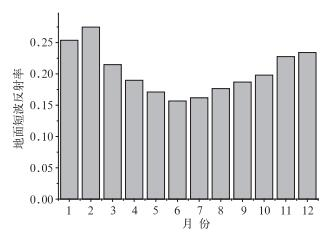图 2 天津地区2009年地面反射率逐月变化 Fig. 2 Monthly variation of ground reflectivity in Tianjin during 2009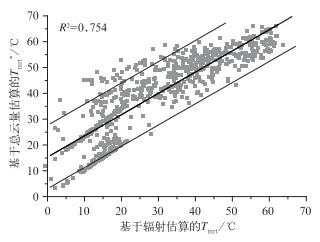图 3 基于辐射估算Tmrt与云量估算Tmrt*的相互关系 Fig. 3 The relationship between Tmrt based on radiation and Tmrt* based on cloud estimation
 $\begin{array}{l} {T_{{\rm{mrt}}}} = {T_{{\rm{mrt}}}}^*\;\;\;\;\;\;\;\;\;\;\;\;\;\;\;\;\;\;\;\;\;\;\;\;\;\;(夜间, N \le 5)\\ {T_{{\rm{mrt}}}} = 1.035{T_{{\rm{mrt}}}}^*-13.425\;\;\;\;\;\;(其他) \end{array}$ (12)

3 讨论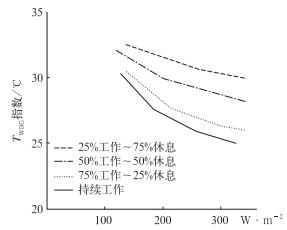图 4 各种劳动/休息时间的TWBG参考曲线 Fig. 4 Reference curves about sorts of labor/rest time TWBG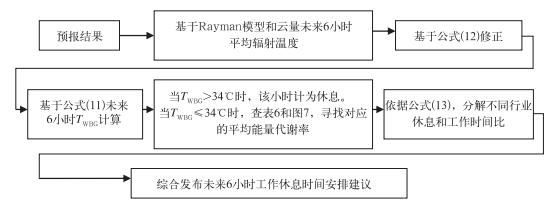图 5 TWBG产品预报和运用流程 Fig. 5 TWBG product forecast and operation process
 $\eta \times 60 = {\eta ^*}t + 32.5\left( {60-t} \right)\\ p = t/\left( {60 - t} \right)$ (13)表 5 能量代谢率分级 Table 5 The classification of energy metabolization rate表 6 天津市区2009年7月23日短时预报结果 Table 6 Short-term forecasting results inTianjin on July 23 2009表 7 2009年7月23日TWBG部分预报结果和建议 Table 7 The forecast results and suggestions for the 23 July 2009 case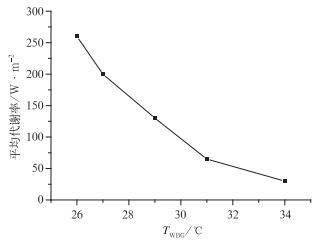图 6 TWBG和平均代谢率关系图TWBG评价指标选择中 Fig. 6 The relation of TWBG and average metabolization rate
4 结语

(1) 通过TWBG与温度、相对湿度、太阳辐射、风速、平均辐射温度的相关关系，分别建立基于常规气象要素和基于平均辐射温度的TWBG指标简化计算方法。

(2) 通过Andreas Matzarakis的方案和RayMan模型，将云量因子引入TWBG回归方程中，实现平均辐射温度的简化计算，建立其与气象预报因子匹配的预报方案。

(3) 根据GB/T 17244-1998和ISO 7243标准体系，将TWBG预报产品运用于环境气象预报，给出未来6小时室外工作安排建议，避免高温条件下室外工作给人体带来的伤害。

  吴兑. 多种人体舒适度预报公式讨论[J]. 气象科技, 2003, 12(31): 370-372.  黄海霞, 李建龙, 黄良美. 南京市小气候日变化规律及其对人体舒适度的影响[J]. 生态学杂志, 2008, 27(4): 601-606.  谈建国, 邵德民, 马雷鸣, 等. 人体热量平衡模型及其在人体舒适度预报中的应用[J]. 南京气象学院学报, 2001, 24(3): 384-390.  张书余. 城市环境气象预报技术[M]. 北京: 气象出版社, 2006: 119-148.  吴结晶, 李瑞光, 穆美舒, 等. 青岛市区夏季暑热指数指数初探[J]. 气象, 2000(4): 33-36. DOI:10.7519/j.issn.1000-0526.2000.04.008  陈莉, 李帅, 周永吉, 等. 用标准有效温度和不舒适指标研究哈尔滨热舒适状况[J]. 气象, 2008, 34(1): 73-79.  杨成芳, 薛德强, 李长军. 山东省人体舒适度区域特征研究[J]. 气象, 2004, 30(10): 7-11. DOI:10.3969/j.issn.1000-0526.2004.10.002  李凤云, 杨玉霞. 体感温度预报方法的改进[J]. 气象, 2002, 28(4): 10-11.  李兴荣, 张小丽, 隋高林, 等. 深圳夏季典型晴天不同小气候区温湿及舒适度特征[J]. 气象, 2010, 36(10): 62-66. DOI:10.7519/j.issn.1000-0526.2010.10.010  张磊, 孟庆林, 赵立华, 等. 室外热环境评价指标湿球黑球温度简化计算方法[J]. 重庆建筑大学学报, 2008, 30(5): 108-117.  钱炜, 唐放鸣. 城市户外环境热舒适度评价模型[J]. 西安建筑科技大学学报, 2001, 33(3): 229-232.  林波荣. 绿化对室外热环境影响的研究[M]. 北京: 清华大学出版社, 2004: 106-127.  Andreas M, Frank R, Helmunt M. Modelling radiation fluxes in simple and complex environments-application of Rayman model[J]. Int J Biometeorol, 2007, 51: 323-334. DOI:10.1007/s00484-006-0061-8  Andreas M, Frank R. Application of Rayman for tourism and climate investigations[J]. Annalen der Meteorologie, 2007, 41(2): 631-636.  余永江. 基于人体与环境热平衡模型的中暑等级预报[D]. 南京: 南京信息工程大学, 2008: 14-16. http://cdmd.cnki.com.cn/Article/CDMD-10300-2008092232.htm  国家技术监督局热环境. 根据TWBG指数(湿球黑球温度)对作业人员热负荷的评价GB/T 17244-1998.